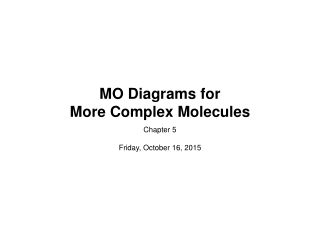Download PresentationMO Diagrams for More Complex Molecules

# MO Diagrams for More Complex Molecules

Download Presentation## MO Diagrams for More Complex Molecules

- - - - - - - - - - - - - - - - - - - - - - - - - - - E N D - - - - - - - - - - - - - - - - - - - - - - - - - - -
##### Presentation Transcript

1. MO Diagrams for More Complex Molecules • Chapter 5 • Friday, October 16, 2015

2. BF3 - Projection Operator Method • boron orbitals • 2s: • A1’ • E’(y) • E’(x) • A1’ • 2py: • E’(y) • A1’ • E’(y) • E’(x) • E’(x) • 2px: • A2’ • E’(y) • E’(x) • A2’’ • 2pz: • A2’’ • E’’(y) • E’’(x)

3. BF3 - Projection Operator Method • boron orbitals • 2s: • A1’ • E’(y) • E’(x) • A1’ • 2py: • E’(y) • A1’ • E’(y) • E’(x) • little overlap • E’(x) • 2px: • A2’ • E’(y) • E’(x) • A2’’ • 2pz: • A2’’ • E’’(y) • E’’(x)

4. –8.3 eV –14.0 eV –18.6 eV –40.2 eV Boron trifluoride • F 2s is very deep in energy and won’t interact with boron. Al Si B Na P Li C S Mg Cl Be N H 3p Al Ar O 3s B 2p F Si 1s P C Ne 2s He S N Cl Ar O F Ne

5. E′ A2′ +E′ –18.6 eV A2″ + E″ Energy A1′ + E′ –8.3 eV a2″ a1′ a2″ e′ A2″ –14.0 eV –40.2 eV A1′ + E′ A1′ Boron Trifluoride σ* σ* π* nb π σ σ nb

6. d orbitals • l= 2, so there are 2l+ 1 = 5 d-orbitals per shell, enough room • for 10 electrons. • This is why there are 10 elements in each row of the d-block.

7. σ-MOs for Octahedral Complexes 1. Point group Oh The six ligands can interact with the metal in a sigma or pi fashion. Let’s consider only sigma interactions for now. • 2. pi sigma

8. σ-MOs for Octahedral Complexes • 2. • 3. Make reducible reps for sigma bond vectors Γσ = A1g + Eg + T1u 4. This reduces to: six GOs in total

9. σ-MOs for Octahedral Complexes Γσ = A1g + Eg + T1u 5. Find symmetry matches with central atom. • Reading off the character table, we see that the group orbitals match the metal s orbital (A1g), the metal p orbitals (T1u), and the dz2and dx2-y2 metal d orbitals (Eg). We expect bonding/antibonding combinations. • The remaining three metal d orbitals are T2g andσ-nonbonding.

10. σ-MOs for Octahedral Complexes • We can use the projection operator method to deduce the shape of the ligand group orbitals, but let’s skip to the results: L6 SALC symmetry label σ1 + σ2 + σ3 + σ4 + σ5 + σ6A1g(non-degenerate) σ1 - σ3 , σ2 - σ4 , σ5 - σ6T1u(triply degenerate) σ1 - σ2 + σ3 - σ4 , 2σ6 + 2σ5 - σ1 - σ2 - σ3 - σ4Eg(doubly degenerate) 5 3 4 2 1 6

11. σ-MOs for Octahedral Complexes • There is no combination of ligand σ orbitals with the symmetry of the metal T2gorbitals, so these do not participate in σ bonding. L + T2g orbitals cannot form sigma bonds with the L6 set. S = 0. T2g are non-bonding

12. σ-MOs for Octahedral Complexes • 6. Here is the general MO diagram for σ bonding in Oh complexes:

13. Summary • MO Theory • MO diagrams can be built from group orbitals and central atom orbitals by considering orbital symmetries and energies. • The symmetry of group orbitals is determined by reducing a reducible representation of the orbitals in question. This approach is used only when the group orbitals are not obvious by inspection. • The wavefunctions of properly-formed group orbitals can be deduced using the projection operator method. • We showed the following examples: homonuclear diatomics, HF, CO, H3+, FHF-, CO2, H2O, BF3, and σ-ML6